# Electronics - Logic Circuit Simplification

### Exercise :: Logic Circuit Simplification - General Questions

6.

Logically, the output of a NOR gate would have the same Boolean expression as a(n):

 A. NAND gate immediately followed by an INVERTER B. OR gate immediately followed by an INVERTER C. AND gate immediately followed by an INVERTER D. NOR gate immediately followed by an INVERTER

Explanation:

No answer description available for this question. Let us discuss.

7.

Which of the examples below expresses the distributive law of Boolean algebra?

 A. A • (B • C) = (A • B) + C B. A + (B + C) = (A • B) + (A • C) C. A • (B + C) = (A • B) + (A • C) D. (A + B) + C = A + (B + C)

Explanation:

No answer description available for this question. Let us discuss.

8.

Which output expression might indicate a product-of-sums circuit construction?

 A.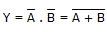B.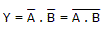C.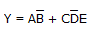D.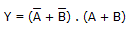Explanation:

No answer description available for this question. Let us discuss.

9.

One of DeMorgan's theorems states that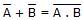. Simply stated, this means that logically there is no difference between:

 A. a NAND gate and an AND gate with a bubbled output B. a NOR gate and an AND gate with a bubbled output C. a NOR gate and a NAND gate with a bubbled output D. a NAND gate and an OR gate with a bubbled output

Explanation:

No answer description available for this question. Let us discuss.

10.

The commutative law of addition and multiplication indicates that:

 A. the way we OR or AND two variables is unimportant because the result is the same B. we can group variables in an AND or in an OR any way we want C. an expression can be expanded by multiplying term by term just the same as in ordinary algebra D. the factoring of Boolean expressions requires the multiplication of product terms that contain like variables